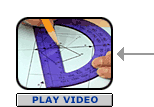# Symmetry Part A: Line Symmetry (30 minutes)

## Session 7, Part A

### In This Part

• Finding Lines of Symmetry
• The Perpendicular Bisector

In Session 5, you saw three ways to move figures around: rotation, reflection, and translation. If you can move an entire design in one of these ways, and that design appears unchanged, then the design is symmetric.

If you can reflect (or flip) a figure over a line and the figure appears unchanged, then the figure has reflection symmetry or line symmetry. The line that you reflect over is called the line of symmetry. A line of symmetry divides a figure into two mirror-image halves. The dashed lines below are lines of symmetry:The dashed lines below are not lines of symmetry. Though they do cut the figures in half, they don’t create mirror-image halves.You can use a Mira (image reflector) or simply the process of cutting and folding to find lines of symmetry. Print the PDF version of the figures above, and compare the lines proposed using a Mira.

In Problems A1 and A2, sketch the figures or print the PDF files of the figures and show the lines of symmetry as dashed lines.

### Problem A1

For each figure, find all the lines of symmetry you can.Problem A2

Find all the lines of symmetry for these regular polygons. Generalize a rule about the number of lines of symmetry for regular polygons.Part A: Finding Lines of Symmetry adapted from IMPACT Mathematics, Course 3, developed by Educational Development Center, Inc. pp. 289-290. © 2000 Glencoe/McGraw-Hill. Used with permission. www.glencoe.com/sec/math

Problems A1 and A2 adapted from IMPACT Mathematics, Course 3, developed by Educational Development Center, Inc. pp. 290-291. © 2000 Glencoe/McGraw-Hill. Used with permission. www.glencoe.com/sec/math

### The Perpendicular Bisector

If point A’ is the mirror image of point A in a figure with a line of symmetry, then the line of symmetry is the perpendicular bisector of the segment AA’.You can use that fact to reflect figures over lines without using a device like a Mira. Suppose you want to reflect the triangle below over the line shown. To reflect point A, draw a segment from A perpendicular to the line. Continue the segment past the line until you’ve doubled its length. You now have point A’, the mirror image of A.Repeat the process for the other two endpoints, and connect them to form the triangle.

### Problem A3

For each figure, reflect the figure over the line shown using perpendicular bisectors. Check your work with a Mira.

Printable PDF of figures### Video Segment

In this video segment, the participants explain different ways in which they came up with perpendicular bisectors to reflect the figures over the line of symmetry.

Did you use the same method as shown in the video segment? Did you come up with a different way of solving the problems?

You can find this segment on the session video approximately 11 minutes and 9 seconds after the Annenberg Media logo.

Problem A3, the preceding text, and the Video Segment problem adapted from IMPACT Mathematics, Course 3, developed by Educational Development Center, Inc. pp. 295-296. © 2000 Glencoe/McGraw-Hill. Used with permission. www.glencoe.com/sec/math

### Problem A1

The isosceles trapezoid has one line of symmetry, the perpendicular bisector of the base. The scalene triangle has no lines of symmetry. The isosceles triangle has one line of symmetry, the perpendicular bisector of the base. The ellipse has two lines of symmetry, one along the major and one along the minor axis. The rectangle has two lines of symmetry, the perpendicular bisector of the longer sides, and the perpendicular bisector of the shorter sides. The circle has infinitely many lines of symmetry, any line going through the center. (Any diameter is a line of symmetry.) The parallelogram pictured has no lines of symmetry. Neither does the trapezoid.### Problem A2

Each regular polygon has as many lines of symmetry as it has sides.### Problem A3